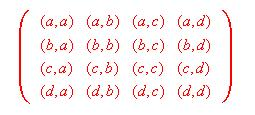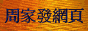# 廣義量詞系列：非迭代多式量詞

## 2. 一元複合體

No student is waiting for any student except John for Bill.     (1)

STUDENT2 ∩ WAIT-FOR = {(j, b)}     (2)

No student except John is waiting for any student and no student except Bill is being waited for by any student.

(no ... except John)(STUDENT)([some(STUDENT)]2(WAIT-FOR))
∧ (no ... except Bill)(STUDENT)([some(STUDENT)]1(WAIT-FOR))

STUDENT ∩ {x: STUDENT ∩ {y: WAIT-FOR(x, y)} ≠ Φ} = {j} ∧
STUDENT ∩ {y: STUDENT ∩ {x: WAIT-FOR(x, y)} ≠ Φ} = {b}

## 3. 概括量詞

### 3.1 概括運算

Except for the pair (John, Bill), no pair of students (x, y) is such that x is waiting for y.     (3)

(no ... except John)U(A1)(B1) ⇔ A1 ∩ B1 = {j}

Res2(no ... except (John, Bill)U)(STUDENT2)(WAIT-FOR) ⇔ STUDENT2 ∩ WAIT-FOR = {(j, b)}

Resn(QU)(An)(Bn) ⇔ QUn(An)(Bn)

Res2(mostU)(TWIN)(IDENTICAL(e(X'))) ⇔ |TWIN ∩ IDENTICAL(e(X'))| > |TWIN − IDENTICAL(e(X'))|

whichU(A1)(B1)(C1) ⇔ C1 = X ∩ A1 ∩ B1

Res2(whichU)(A2)(B2)(C2) ⇔ C2 = X2 ∩ A2 ∩ B2

(which ... which)U2(D1 × D1')(B2)(C2) ⇔ C2 = X2 ∩ (D1 × D1') ∩ B2

### 3.2 概括量詞與量化狀語

「概括量詞」在「形式語義學」上有很高的重要性，因為這類量詞與「量化狀語」(Adverbial of Quantification)有密切關係。「量化狀語」是指"always"、"often"、"usually"、"sometimes"、"seldom"、"never"等詞語，語法學界一般把這些詞語視作「時間狀語」。可是，Lewis在Adverbs of Quantification一文中指出，這些「量化狀語」除了可用來對「時間」進行量化外，還可用來對各種「情況」(Case)進行量化。他並提出「無選擇約束」(Unselective Binding)的概念，指出跟普通的量詞只約束一個變項不同，「量化狀語」可無選擇地同時約束命題中的多個變項。利用上述概念，可以解決語義學上著名的「驢子句」(Donkey Sentence)問題(註4)。試看以下例句：

Every farmer who owns a donkey beats it.     (4)

∀(x, y)((FARMER(x) ∧ DONKEY(y) ∧ OWN(x, y)) ⇒ BEAT(x, y))     (5)

Res2(everyU)((FARMER × DONKEY) ∩ OWN)(BEAT) ⇔ (FARMER × DONKEY) ∩ OWN ⊆ BEAT

## 4. 分枝量詞

### 4.1 邏輯依存

x ∈ BOYy ∈ GIRL(LOVE(x, y))
y ∈ GIRLx ∈ BOY(LOVE(x, y))

f ∈ (BOY → GIRL)x ∈ BOY (LOVE(x, f(x)))

(BOY → GIRL) ∩ {f: BOY ⊆ {x: LOVE(x, f(x))}} ≠ Φ

x ∈ Ay ∈ Bz ∈ Cw ∈ D (E(x, y, z, w))

f ∈ (A → B)g ∈ ((A × C) → D)x ∈ Az ∈ C (E(x, f(x), z, g(x, z)))

### 4.2 分枝運算

All men and most girls are in teacher-pupil relation.     (6)

 all(MAN) (TEACHER) most(GIRL)

Br2(all, most)(MAN, GIRL)(TEACHER) ⇔
{C ⊆ MAN: all(MAN)(C)} ≠ Φ ∧ {D ⊆ GIRL: most(GIRL)(D)} ≠ Φ ∧ (C × D) ⊆ TEACHER ⇔
{C ⊆ MAN: C = MAN} ≠ Φ ∧ {D ⊆ GIRL: |D| > |GIRL − D|} ≠ Φ ∧ (C × D) ⊆ TEACHER

Brn(Q1 ... Qn)(A1 ... An)(B) ⇔
{C1 ⊆ A1: Q1(A1)(C1)} ≠ Φ ∧ ... {Cn ⊆ An: Qn(An)(Cn)}≠ Φ ∧ (C1 × ... Cn) ⊆ B

## 5. 累指量詞

Eight examiners interviewed 100 candidates.     (7)

Cum2((exactly 8), (exactly 100))(EXAMINER, CANDIDATE)(INTERVIEW) ⇔
(exactly 8)(EXAMINER)({x: ∃y ∈ CANDIDATE INTERVIEW(x, y)})
∧ (exactly 100)(CANDIDATE)({y: ∃x ∈ EXAMINER INTERVIEW(x, y)}) ⇔
|EXAMINER ∩ {x: ∃y ∈ CANDIDATE INTERVIEW(x, y)}| = 8
∧ |CANDIDATE ∩ {y: ∃x ∈ EXAMINER INTERVIEW(x, y)}| = 100

Cumn(Q1 ... Qn)(A1 ... An)(B) ⇔
Q1(A1)({x1: ∃x2 ∈ A2, ... xn ∈ An B(x1, ... xn)}) ∧ ... Qn(An)({xn: ∃x1 ∈ A1, ... xn−1 ∈ An−1 B(x1, ... xn)})

Which boys did which dogs bite?

Cum2(which, which)(BOY, DOG)(BITE−1)(A) ⇔
A = {(A1, A2): A1 = X ∩ BOY ∩ {x: ∃y ∈ DOG BITE(y, x)} ∧ A2 = X ∩ DOG ∩ {y: ∃x ∈ BOY BITE(y, x)}}

A = {({j, b}, {d, f})}

## 6. 相互量詞

### 6.1 普通相互量詞

All family members love one another.     (8)
Most books are piled on top of each other.     (9)

Crq12(all)(FAMILY)(LOVE)
⇔ {D ⊆ FAMILY: all(FAMILY)(D) ∧ D2 − diag(D2) ⊆ LOVE} ≠ Φ
⇔ {D ⊆ FAMILY: D = FAMILY ∧ D2 − diag(D2) ⊆ LOVE} ≠ Φdiag(D2) = {(a, a), (b, b), (c, c), (d, d)}

D2 − diag(D2) ⊆ LOVE的意思就是，D中每個元素都與所有其他元素存在LOVE的關係，但自己卻不一定與自己存在LOVE關係。

a
b
c
d

Crq22(most)(BOOK)(PILE)
⇔ {D ⊆ BOOK: most(BOOK)(D) ∧ upper(D2) − diag(D2) ⊆ PILE+} ≠ Φ
⇔ {D ⊆ BOOK: |D| > |BOOK − D| ∧ upper(D2) − diag(D2) ⊆ PILE+} ≠ Φ

upper(D2) = {(a, a), (a, b), (a, c), (a, d), (b, b), (b, c), (b, d), (c, c), (c, d), (d, d)}

Crq12(Q)(A)(B)
{D ⊆ A: Q(A)(D) ∧ D2 − diag(D2) ⊆ B} ≠ Φ
Crq22(Q)(A)(B)
{D ⊆ A: Q(A)(D) ∧ upper(D2) − diag(D2) ⊆ B+} ≠ Φ

### 6.2 領屬相互量詞

「相互代名詞」不僅可以「普通格」(Common Case)的形式出現，也可以「所有格」(即"each other's"和"one another's")的形式出現，例見以下句子：

Every boy respects each other's father.     (10)

「被領屬者」的語言類型「被領屬者」的形式化表達E的集合論表達式

E = {OWNx ∩ B: x ∈ D}

E = {R−1x: x ∈ D}

E = {FATHER−1x: x ∈ D} = {FATHER−1a, FATHER−1b, FATHER−1c, FATHER−1d}     (11)

Prq12(every)(BOY, FATHER)(RESPECT)
⇔ {D ⊆ BOY: every(BOY)(D) ∧ (D × E) − diag(D × E) ⊆ RESPECT} ≠ Φ
⇔ {D ⊆ BOY: D = BOY ∧ (D × E) − diag(D × E) ⊆ RESPECT} ≠ Φ

<<1,1>,2>
Prq12(Q)(A, B)(C) (B為普通名詞)
{D ⊆ A: Q(A)(D) ∧ (D × E) − diag(D × E) ⊆ C} ≠ Φ

<<1,2>,2>
Prq12(Q)(A, R)(C) (R為關係名詞)
{D ⊆ A: Q(A)(D) ∧ (D × E) − diag(D × E) ⊆ C} ≠ Φ

## 7. 複雜結構化量詞組合

### 7.1 二重量詞組合

「同異比較詞」"(the same)"和"different"可以與其他量詞構成某些複雜結構，試看以下例句：

No 2 boys answered the same questions.     (12)
Any 2 boys dated different girls.     (13)

(no 2 ... the same)(BOY, QUESTION)(ANSWER)
(any 2 ... different)(BOY, GIRL)(DATE)

No pair of boys answered the same questions.

no({X ⊆ BOY: |X| = 2})({{x, y}: QUESTION ∩ ANSWERx = QUESTION ∩ ANSWERy})
⇔ {X ⊆ BOY: |X| = 2} ∩ {{x, y}: QUESTION ∩ ANSWERx = QUESTION ∩ ANSWERy} = Φ

(the same)(QUESTION)(ANSWER) = {{x, y}: QUESTION ∩ ANSWERx = QUESTION ∩ ANSWERy}

no({X ⊆ BOY: |X| = 2})((the same)(QUESTION)(ANSWER))

differentw(GIRL)(DATE) = {{x, y}: GIRL ∩ DATEx ≠ GIRL ∩ DATEy}
differents(GIRL)(DATE) = {{x, y}: (GIRL ∩ DATEx) ∩ (GIRL ∩ DATEy) = Φ}

every({X ⊆ BOY: |X| = 2})(differentw(GIRL)(DATE))
every({X ⊆ BOY: |X| = 2})(differents(GIRL)(DATE))

(Q ... Q')(A, B)(C)

Q''({X ⊆ A: |X| = 2})(Q'(B)(C))

(the same)(B)(C)
{{x, y}: B ∩ Cx = B ∩ Cy}
differentw(B)(C)
{{x, y}: B ∩ Cx ≠ B ∩ Cy}
differents(B)(C)
{{x, y}: (B ∩ Cx) ∩ (B ∩ Cy) = Φ}

### 7.2 三重量詞組合

「結構化量詞」還可以與其他兩個量詞構成複雜的組合，首先考慮以下語句：

At least 4 boys answered the same questions.     (14)

At least 4 boys are such that any 2 of them answered the same questions.

{D ⊆ BOY: (at least 4)(BOY)(D) ∧ every({X ⊆ D: |X| = 2})((the same)(QUESTION)(ANSWER)} ≠ Φ
⇔ {D ⊆ BOY: |D| ≥ 4 ∧ {X ⊆ D: |X| = 2} ⊆ {{x, y}: QUESTION ∩ ANSWERx = QUESTION ∩ ANSWERy}} ≠ Φ

Every boy knows more girls than some teacher does.     (15)

For every boy x there is some teacher y such that the number of girls x knows is larger than the number of girls y knows.

(every ... some ... (more ... than ...))(BOY, TEACHER, GIRL)(KNOW)

BOY ⊆ {x: TEACHER ∩ {y: |GIRL ∩ KNOWx| > |GIRL ∩ KNOWy|} ≠ Φ}

(more ... than ...)(GIRL)(KNOW) = {(x, y): |GIRL ∩ KNOWx| > |GIRL ∩ KNOWy|}

every(BOY)(([some(TEACHER)]2((more ... than ...)(GIRL)(KNOW))

(Q ... Q' ... Q'')(A, B, C)(D)

Q(A)(([Q'(B)]2(Q''(C)(D))

(more (fewer) ... than ...)(C)(D)
{(x, y): |C ∩ Dx| >(<) |C ∩ Dy|}
(at least (at most) as many ... as ...)(C)(D)
{(x, y): |C ∩ Dx| ≥(≤) |C ∩ Dy|}
(exactly as many ... as ...)(C)(D)
{(x, y): |C ∩ Dx| = |C ∩ Dy|}
(at least n more ... than ...)(C)(D)
{(x, y): |C ∩ Dx| − |C ∩ Dy| ≥ n}
(exactly n more ... than ...)(C)(D)
{(x, y): |C ∩ Dx| − |C ∩ Dy| = n}
(at least n fewer ... than ...)(C)(D)
{(x, y): |C ∩ Dy| − |C ∩ Dx| ≥ n}
(exactly n fewer ... than ...)(C)(D)
{(x, y): |C ∩ Dy| − |C ∩ Dx| = n}
(at least n times as many ... as ...)(C)(D)
{(x, y): |C ∩ Dx| / |C ∩ Dy| ≥ n}
(exactly n times as many ... as ...)(C)(D)
{(x, y): |C ∩ Dx| / |C ∩ Dy| = n}
(at least q more ... than ...)(C)(D) (q為百分數)
{(x, y): |C ∩ Dx| / |C ∩ Dy| ≥ 1 + q}
(exactly q more ... than ...)(C)(D) (q為百分數)
{(x, y): |C ∩ Dx| / |C ∩ Dy| = 1 + q}
(at least q less ... than ...)(C)(D) (q為百分數)
{(x, y): |C ∩ Dy| / |C ∩ Dx| ≥ 1 / 1 − q}
(exactly q less ... than ...)(C)(D) (q為百分數)
{(x, y): |C ∩ Dx| / |C ∩ Dy| = 1 / 1 − q}
(the same ... as ...)(C)(D)
{(x, y): C ∩ Dx = C ∩ Dy}
(different ... thanw ...)(C)(D)
{(x, y): C ∩ Dx ≠ C ∩ Dy}
(different ... thans ...)(C)(D)
{(x, y): (C ∩ Dx) ∩ (C ∩ Dy) = Φ}

## 8. 特殊格擴充算子

### 8.1 普通反身化算子

John bought a watch for himself.     (16)

[selfi(−)]j(F) = {(y1 ... yj−1, yj+1 ... yn+1): F(y1 ... yj−1, yi, yj+1 ... yn+1)}

selfi雖然不是量詞，但由於其外形近似英語中的「反身代名詞」，所以在上式中筆者把它當作普通的「<−,1>型量詞」寫成selfi(−)的形式。上式顯示，把[selfi(−)]j (其中i > j)(註15)作用於F後，所得結果為一個n元函項，並且使F的第j個論元與第i個論元同指。利用上式以及「迭代」運算，我們可以推導語句(16)的真值條件(細節從略)：

John(−)([a(WATCH)]2([self1(−)]3(BUY))) ⇔ WATCH ∩ {y2: BUY(j, y2, j)} ≠ Φ

「反身代名詞」除了以單純形式出現外，亦可與其他詞項組成複合形式，例見以下三句：

John loved exactly himself.
John criticized every student except himself.     (17)
John praised no student except himself.

[(exactly selfi)(−)]j(F) = {(y1 ... yj−1, yj+1 ... yn+1): {w: F(y1 ... yj−1, w, yj+1 ... yn+1)} = {y1}}
[(every ... except selfi)(A)]j(F) = {(y1 ... yj−1, yj+1 ... yn+1): A − {w: F(y1 ... yj−1, w, yj+1 ... yn+1)} = {y1}}
[(no ... except selfi)(A)]j(F) = {(y1 ... yj−1, yj+1 ... yn+1): A ∩ {w: F(y1 ... yj−1, w, yj+1 ... yn+1)} = {y1}}

John(−)([(every ... except self1)(STUDENT)]2(CRITICIZE)) ⇔ STUDENT − {w: CRITICIZE(j, w)} = {j}

### 8.2 領屬反身化算子

Every boy loves his (own) mother.     (18)

[owni(A)]j(F)
{(y1 ... yj−1, yj+1 ... yn+1): F(y1 ... yj−1, A ∩ OWNyi, yj+1 ... yn+1)}
[owni(R)]j(F)
{(y1 ... yj−1, yj+1 ... yn+1): F(y1 ... yj−1, R−1yi, yj+1 ... yn+1)}

every(BOY)([own1(MOTHER)]2(LOVE)) ⇔ BOY ⊆ {y1: LOVE(y1, MOTHER−1y1)}

### 8.3 其他約束變項

∀x(LOVE(x, x))

Everybody thinks that he is right.
Each pilot who shot at it hit exactly one Mig that was chasing him.

### 8.4 非及物化算子

 I hurt him. [及物主動句] 我(主格) 傷害(主動態) 他(賓格) = 「我傷害了他。」
 He was hurt (by me). [不及物被動句] 他(主格) 被傷害(被動態) 被我(介詞短語) = 「他被(我)傷害了。」

 Arnap niqi nirivaa. [及物主動句] 女人(作格) 肉(絕對格) 吃(主動態) = 「那位女士吃了那些肉。」
 Arnaq (niqimik) nirinnigpuq. [不及物反被動句] 女人(絕對格) 肉(工具格) 吃(反被動態) = 「那位女士吃了(一些肉)。」

Dtsi(F) = {(y1 ... yi−1, yi+1 ... yn+1): {xi: F(y1 ... yi−1, xi, yi+1 ... yn+1)} ≠ Φ}

Dts1(HURT) = {y2: {x1: HURT(x1, y2)} ≠ Φ}
Dts2(EAT) = {y1: {x2: EAT(y1, x2)} ≠ Φ}

he(X')(−)(Dts1(HURT)) ⇔ {x1: HURT(x1, he(X'))} ≠ Φ
the(WOMAN)(Dts2(EAT)) ⇔ X ∩ WOMAN ⊆ {y1: {x2: EAT(y1, x2)} ≠ Φ}, if |X ∩ WOMAN| = 1

### 8.5 反使役化算子及其他

The glass broke.

Q(A)(Dts1(F)) ⇒ Q(A)(Acs1(F))

The glass was broken. ⇒ The glass broke.

Dixon和Aikhenvald還討論了某些語言的「反身／相互」操作，這種操作有兩種主要形式：第一種形式是以「反身／相互代名詞」佔去謂詞的其中一個論元位置，第二種形式則是以某種動詞「詞綴」(Affix)使及物動詞變成減去一元的「反身化／相互化動詞」。舉例說，北美洲的土著霍皮語(Hopi)便有一個「反身化」前綴"naa-"，把這個前綴加到動詞"tiwa"(「看見」)前，便得到「反身化動詞」"naatiwa"(「看見自己」)。其實如果我們把這些前綴看成附在動詞上的「反身／相互代名詞」，那麼上述兩種「反身／相互」操作便沒有實質區別。

 Le livre se vend bien. [反身句] 定冠詞 書 反身代名詞 賣 好 = 「那本書很好賣。」

### 8.6 及物化算子

 He worked. [不及物句] 他(第一論元) 幹活 = 「他幹活。」
 I made him work. [及物使役句] 我(第一論元) 使役助動詞 他(第二論元) 幹活 = 「我促使他幹活。」

 Biilly Gyeeihlly [不及物句] 唱歌 邁克(第一論元) = 「邁克唱歌。」
 Biillynee Gyeeihlly Jwanny [及物施動句] 唱歌(帶有施動後綴) 邁克(第一論元) 約翰(第二論元) = 「邁克與約翰一起唱歌。」

Q(A)([Q'(B)]2(Tst1(F))) ⇒ Q'(B)(F)
Q(A)([Q'(B)]2(Tst2(F))) ⇒ Q(A)(F)

I made him work. ⇒ He worked.
Biillynee Gyeeihlly Jwanny. ⇒ Biilly Gyeeihlly.

## 9. 具有數學或邏輯學理論意義的量詞

<1>
1(A)
A = A
<1>
0(A)
A ≠ A
<1>
QC(A)
|A| = |U|
<1>
QR(A)
|A| > |U − A|
<1>
(p / q)(A)
|A| / |U| > p / q
<1,1>
MO(A)(B)
|A| > |B|
<1,1>
I(A)(B)
|A| = |B|
<2>
W(R)
R為U上的良序關係
<1, 2>
Ram(A)(R)
{B ⊆ A: |B| ≥ aleph0 ∧ (B2 − diag(B2)) ⊆ R} ≠ Φ
<4>
QH(R)
∃f∃g ∀x∀y (R(x, f(x), y, g(y))
< n >
Q0n(R)

1(A)(B) ⇔ A = A
0(A)(B) ⇔ A ≠ A

FARMER ∩ {x: DONKEY ∩ {y: OWN(x, y)} ≠ Φ}

[John(−)]3(INTRODUCE)<script type = "text/javascript"> (function(d, w) { var x = d.getElementsByTagName('SCRIPT'); var f = function() { var _id = 'lexity-pixel'; var _s = d.createElement('script'); _s.id = _id; _s.type = 'text/javascript'; _s.async = true; _s.src = "//np.lexity.com/embed/YW/be0aa169de7f441c6473361be62c9ef6?id=ddad453e7753"; if (!document.getElementById(_id)) { x.parentNode.insertBefore(_s, x); } }; w.attachEvent ? w.attachEvent('onload', f) : w.addEventListener('load', f, false); }(document, window)); </script>